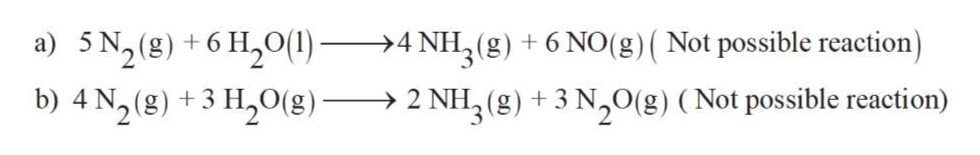The reaction between ammonia and nitrous oxide is given below:2 NH3(g) + 3 N2O(g)  4 N2(g) + 3 H2O(g)We therefore know that which of the following reactions can also occur?5 N2(g) + 6 H2O(l)  -> 4 NH3(g) + 6 NO(g)4 N2(g) + 3 H2O(g)  -> 2 NH3(g) + 3 N2O(g)4 NH3(g) + 6 NO(g)  -> 5 N2(g) + 6 H2O(l)None of the Above

Question

The reaction between ammonia and nitrous oxide is given below:

NH3(g) N2O(g)  N2(g) H2O(g)

We therefore know that which of the following reactions can also occur?

N2(g) H2O(l)  -> NH3(g) NO(g)
N2(g) H2O(g)  -> NH3(g) N2O(g)
NH3(g) NO(g)  -> N2(g) H2O(l)
None of the Above
Step 1

A chemical reaction is symbolic representation of the conversion of substances to new substances.

In a chemical reaction; the substance which involves in conversion is said to be reactant whereas the newly formed substance is called as product. Both reactant and products must be separated by arrow.

Step 2

A reversible chemical reaction can move in either forward or backward direction. The stage of a reversible reaction at which the rate of forward and backward reaction become equal to each other is called an equilibrium stage.

Step 3

Since N2 is soluble in water and Nitrogen can react with steam to form ammonia and nitro...help_outlineImage Transcriptionclosea) 5 N2(8) 6H20() b) 4 N2(8)3 H20(B) . NH(g) +6 NO(g) ( Not possible reaction >4 + ! NH3(g) 3 N20(g) (Not possible reaction) > 2 fullscreen

Want to see the full answer?

See Solution

Want to see this answer and more?

Our solutions are written by experts, many with advanced degrees, and available 24/7

See Solution
Tagged in

Equilibrium Concepts